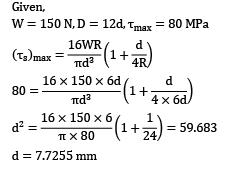Courses

# Test:Design Of Springs (Level - 2)

## 20 Questions MCQ Test Machine Design | Test:Design Of Springs (Level - 2)

Description
This mock test of Test:Design Of Springs (Level - 2) for Mechanical Engineering helps you for every Mechanical Engineering entrance exam. This contains 20 Multiple Choice Questions for Mechanical Engineering Test:Design Of Springs (Level - 2) (mcq) to study with solutions a complete question bank. The solved questions answers in this Test:Design Of Springs (Level - 2) quiz give you a good mix of easy questions and tough questions. Mechanical Engineering students definitely take this Test:Design Of Springs (Level - 2) exercise for a better result in the exam. You can find other Test:Design Of Springs (Level - 2) extra questions, long questions & short questions for Mechanical Engineering on EduRev as well by searching above.
QUESTION: 1

Solution:
QUESTION: 2

### A body weighing 1000 kg falls 8 cm and strikes a 500 kg/cm spring. The deformation of spring will be __________ cm.

Solution: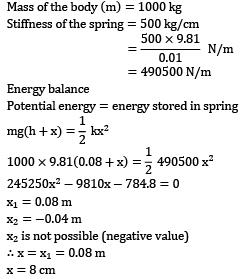QUESTION: 3

### Figure given above shows as spring-mass system where the mass m is fixed in between two springs of stiffness d . What is the equivalent spring stiffness?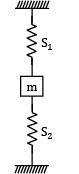Solution:

The arrangement is a parallel combination.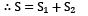QUESTION: 4

A closed-coil helical spring is subjected to a torque about its axis. The spring wire would experience a

Solution:
QUESTION: 5

The maximum shear stress occurs on the outermost fibers of a circular shaft under torsion. In a close coiled helical spring, The maximum shear stress occurs on the

Solution: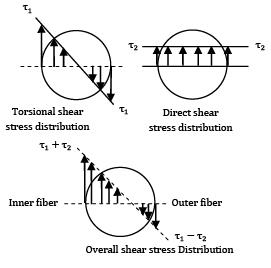QUESTION: 6

A closely coiled spring of 20 cm mean diameter is having 25 coils of 2 cm diameter. Modulus of rigidity of the material is 107 N/cm2 . Stiffness of spring is

Solution:

Given,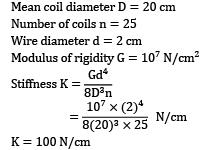QUESTION: 7

A closed coil helical spring having 10 active turns is made of 8 mm diameter steel wire. The mean coil diameter is 10 cm. If G = 80 GPa for the material of the spring, the extension of the spring under the tensile load of 200 N will be

Solution: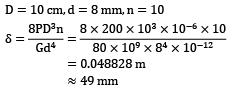QUESTION: 8

A close-coiled helical spring is made of 5 mm diameter wire coiled to 50 mm mean diameter. Maximum shear stress in the spring under the action of an axial force is 20 N/mm2. The maximum shear stress in a spring made of 3 mm diameter wire coiled to 30 mm mean diameter, under the action of the same force will be nearly

Solution: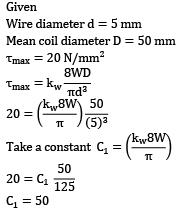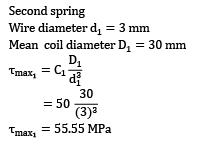QUESTION: 9

A closely-coiled helical spring is made of 10 mm diameter steel wire, with the coil consisting of 10 turns with a mean diameter 120 mm. The spring carries an axial pull of 200 N. What is the value of shear stress induced in the spring neglecting the effect of stress concentration and of deflection in the spring, when the modulus of rigidity is 80 kN/mm2?

Solution: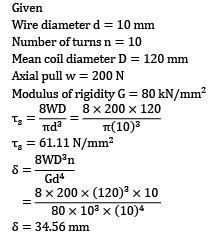QUESTION: 10

A closely-coiled helical spring is acted upon by an axial force. The maximum shear stress developed in the spring is t. Half of the length of the spring is cut off and the remaining spring is acted upon by the same axial force. The maximum shear stress in the spring in new condition will be

Solution: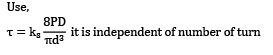QUESTION: 11

A closed coil helical spring has 15 coils. If five coils of this spring are removed by cutting, the stiffness of the modified spring will

Solution: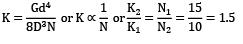QUESTION: 12

A block of weight 2 N falls from a height of 1 m on the top of a spring. If the spring gets compressed by 0.1 m to bring the weight momentarily to rest, then the spring constant would be

Solution:

Kinetic energy of block = potential energy of spring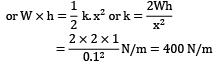QUESTION: 13

The springs of a chest expander are 60 cm long when unstretched. Their stiffness is . The work done in stretching them to 100 cm is

Solution: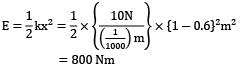QUESTION: 14

A spring of stiffness ‘k’ is extended from a displacement to a displacement the work done by the spring is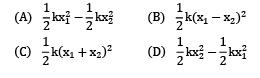Solution: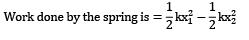*Answer can only contain numeric values
QUESTION: 15

A load of 2 kN is dropped axially on a close coiled helical spring from a height of 250 mm. The spring has 20 effective turns, and it is made of 25 mm diameter wire. The spring index is 8. The amounts of compression produced is 290 mm, the modulus of rigidity for the material of the spring is 84 kN/mm2. The maximum shear stress induced in the spring is __________ MPa.

(A) 285.5

(B) 286.5

Solution: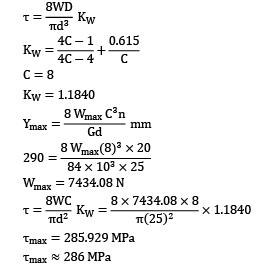QUESTION: 16

A railway wagon moving at a velocity of . is brought to rest by a bumper consisting of two helical springs arranged in parallel. Mass of wagon is 1500 kg. The springs are compressed by 150 mm in bringing the wagon to rest. Spring index is 6. Ultimate tensile strength is and permissible shear stress for spring wire is 50% of ultimate tensile strength is 1250 N/mm2. What is wire diameter?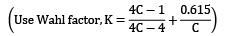Solution: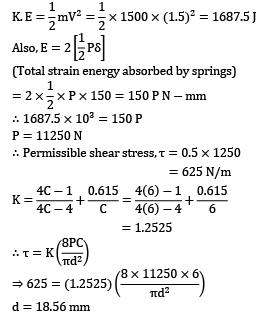*Answer can only contain numeric values
QUESTION: 17

The inner spring of a compound helical spring is concentric with the outer one but is 8 mm shorter than that. The outer spring is of 30 mm mean diameter, has 12 coils with a wire diameter of 4 mm. If an axial load of 250 N compresses the outer spring by 20 mm, the stiffness of the inner spring is __________ N/mm.

(A) 8

(B) 8

Solution: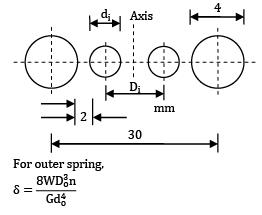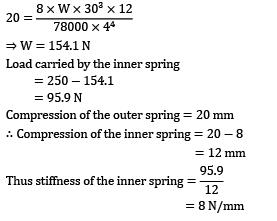QUESTION: 18

A helical tension spring, which is fixed to a rigid support at one end and carries weight at other free end. The pointer attached to the free end shows the reading of 83 mm. The maximum capacity of spring balance is 498 N. The spring index is 5. The ultimate tensile strength of spring material is 1350 MPa. The permissible shear stress for spring material can be taken as 75% of the ultimate tensile strength. Modulus of rigidity for material is 75750 N/mm2. If curvature effect is negligible and wire diameter available are in the range of 1, 2, 3, 4 and 5 mm and the number of coil is 38. What is the actual spring rate of spring?

Solution: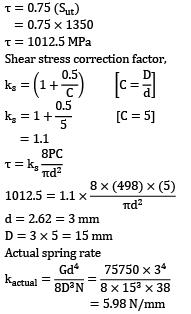QUESTION: 19

Assertion (A): Concentric cylindrical helical springs which are used to have greater spring force in a limited space is wound in opposite directions.

Reason (R): Winding in opposite directions prevents locking of the two coils in case of misalignment or buckling.

Solution:
QUESTION: 20

A closed-coil helical spring is to carry a load of 150 N. The mean coil diameter has to be 12 times that of the wire diameter. If maximum shear stress should not exceed 80 MPa after considering the effect of direct stress, the diameter of the wire will be __________ mm.

(A) 7.65

(B) 7.80

Solution: